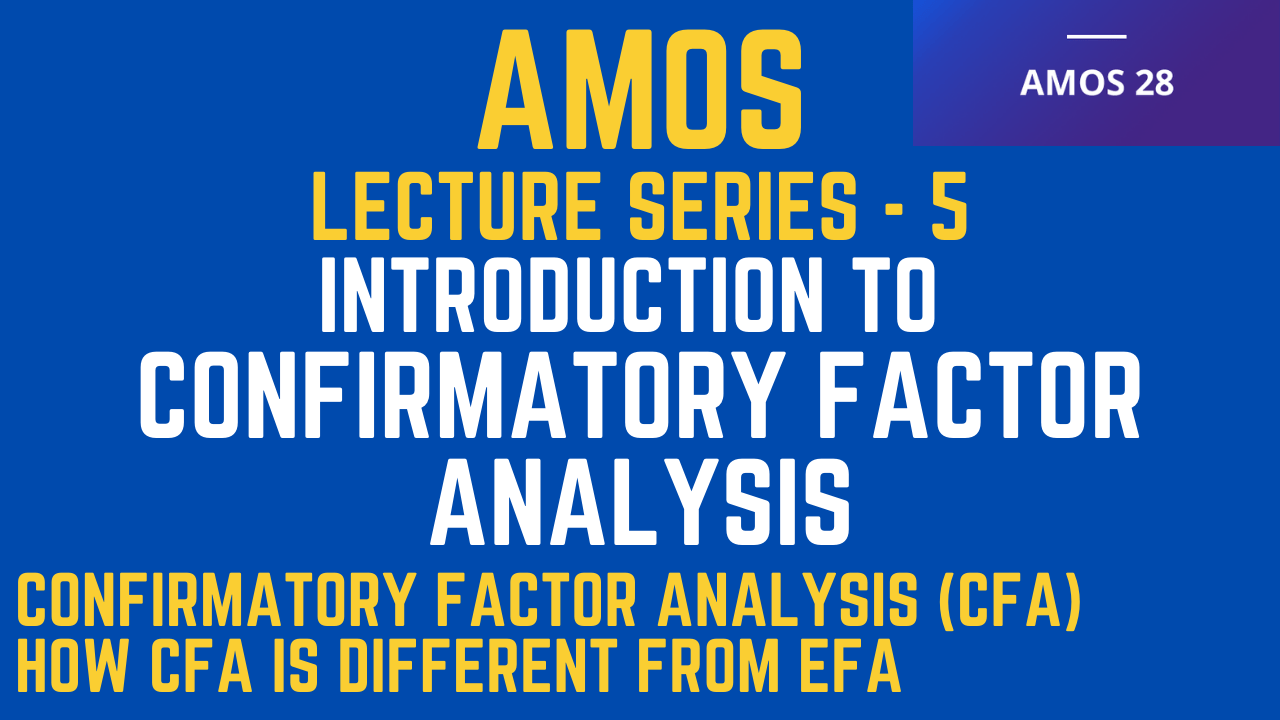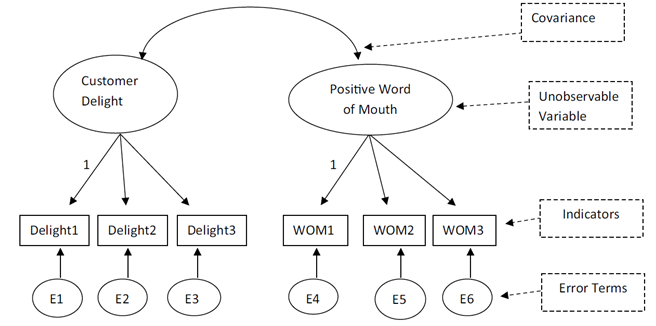# Introduction to Confirmatory Factor Analysis

## Confirmatory Factor Analysis

The session will discuss

• Confirmatory Factor Analysis (CFA)
• How CFA is Different from EFA### IBM SPSS AMOS Lecture Series

Lecture 5 of the IBM SPSS AMOS tutorial series.

## Confirmatory Factor Analysis (CFA)

• Confirmatory factor analysis (CFA) is a statistical technique that analyzes how well your indicators measure your unobserved constructs and if your unobserved constructs are uniquely different from one another.
• In a CFA, an unobservable construct is often referred to as a “factor”.
• So, when we use the term “factor”, it represents an unobservable construct we are trying to measure.
• In a diagram format, an unobserved variable is represented by a circle or oval.
• The indicators that measure the unobserved variable will have single headed arrows coming from the unobserved construct to each of the indicators.
• Each indicator is represented by a square or a rectangle.
• The single arrow line directly from the factor, or unobservable construct, to the indicator represents the influence that is reflected from the unobservable construct to its indicators.
• Statistical estimates of these direct effects are called “factor loadings” and are interpreted as regression coefficients that may be in unstandardized or standardized form.
• Indicators that “reflect” an unobservable construct or factor are called simply reflective indicators.
• With most indicators, a level of error is present. Each measurement error of an indicator represents “unique variance”, or variance not explained by the factor level.
• Measurement errors are represented by two types of unique variance: random error and systematic error.
• Random error occurs when the measurement of a concept is unpredictably fluctuating due to unforeseen or random influences. This type of error is considered “noise” because there is often no consistency to the measurement error.
• Systematic error is different in that the error is systematic in nature. The results of a measurement can be consistently high or low due to a systematic influence in the measurement of a concept.
• For instance, taking a survey online as compared to a paper and pencil survey may systematically influence the way respondents answer questions about a construct. With systematic error, this is often classified as a “bias” compared to the “noise” of random error. Note that if an unobserved variable has only one indicator, then the error term for the single indicator variable is constrained to have a mean of 0 and a variance of 0. (You must assume the indicator has no measurement error—which is often a very flawed assumption.)
• In a CFA, you also want to account for “unmeasured covariance”. To do this, a two-headed arrow is drawn between all independent unobservable variables.
• In a CFA, the analysis will treat all unobserved variables as exogenous or independent variables.
• Thus, you will draw a covariance double headed arrow between each unobservable construct in a CFA.
• If you don’t do this, AMOS will give you an error message stating that a covariance should be drawn between all unobserved constructs in your CFA.Source: Collier, J. E. (2020). Applied structural equation modeling using AMOS: Basic to advanced techniques. Routledge.

## How CFA is different from EFA?

• An exploratory factor analysis (EFA) is useful in data reduction of a large number of indicators and can be quite helpful in seeing if indicators are measuring more than one construct.
• EFAs are typically the first step in determining if an indicator is measuring a construct. In an EFA, the researcher is not denoting which indicators are measuring a construct.
• The analysis simply tries to let every indicator load on every construct. This is where “cross loading” can occur, where an indicator is actually loading strongly on more than one construct. This is a concern that your indicator is not a strong measure of the specified construct.
• In contrast, a CFA does not allow an indicator to load on more than one construct. The researcher prior to the analysis will specify what the indicators are for each construct, and those indicators can load only on that specific construct.
• Other ways that an EFA and CFA are different is an EFA is typically conducted with correlation matrices, which can be problematic in comparing parameters across samples.
• CFA uses a covariance matrix and is more adept at handling comparisons across samples.
• An EFA will also consider data rotation that is often done to achieve a better loading of indicators on a construct or sometimes a reduction of cross loading with other constructs.
• A CFA does not worry about rotation because you are denoting the specific items that are loading on a construct.

## References

• Collier, J. E. (2020). Applied structural equation modeling using AMOS: Basic to advanced techniques. Routledge.
• Awang, Z. (2015). A Handbook on SEM (2nd Edition). Malaysia